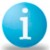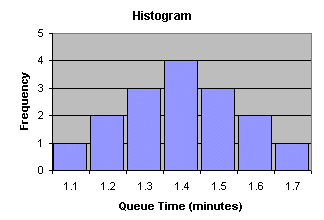# Histogram

ByKerri Simon

### Purpose of a Histogram

A histogram is used to graphically summarize and display the distribution of a process data set.Sample Histogram Depiction

### How to Construct a Histogram

A histogram can be constructed by segmenting the range of the data into equal sized bins (also called segments, groups or classes). For example, if your data ranges from 1.1 to 1.8, you could have equal bins of 0.1 consisting of 1 to 1.1, 1.2 to 1.3, 1.3 to 1.4, and so on.

The vertical axis of the histogram is labeled Frequency (the number of counts for each bin), and the horizontal axis of the histogram is labeled with the range of your response variable.

You then determine the number of data points that reside within each bin and construct the histogram. The bins size can be defined by the user, by some common rule, or by software methods (such as Minitab).

### What Questions the Histogram Answers

• What is the most common system response?
• What distribution (center, variation and shape) does the data have?
• Does the data look symmetric or is it skewed to the left or right?
• Does the data contain outliers?